login  home  contents  what's new  discussion  bug reports help  links  subscribe  changes  refresh  edit

Refs:

We need the Axiom LinearOperator library.

fricas
(1) -> )library CARTEN ARITY CMONAL CPROP CLOP CALEY
CartesianTensor is now explicitly exposed in frame initial
CartesianTensor will be automatically loaded when needed from
/var/aw/var/LatexWiki/CARTEN.NRLIB/CARTEN
Arity is now explicitly exposed in frame initial
Arity will be automatically loaded when needed from
/var/aw/var/LatexWiki/ARITY.NRLIB/ARITY
ClosedMonoidal is now explicitly exposed in frame initial
ClosedMonoidal will be automatically loaded when needed from
/var/aw/var/LatexWiki/CMONAL.NRLIB/CMONAL
ClosedProp is now explicitly exposed in frame initial
ClosedProp will be automatically loaded when needed from
/var/aw/var/LatexWiki/CPROP.NRLIB/CPROP
ClosedLinearOperator is now explicitly exposed in frame initial
ClosedLinearOperator will be automatically loaded when needed from
/var/aw/var/LatexWiki/CLOP.NRLIB/CLOP
CaleyDickson is now explicitly exposed in frame initial
CaleyDickson will be automatically loaded when needed from
/var/aw/var/LatexWiki/CALEY.NRLIB/CALEY

Use the following macros for convenient notation

fricas
-- summation
macro Σ(x,i,n)==reduce(+,[x for i in n])
Type: Void
fricas
-- list
macro Ξ(f,i,n)==[f for i in n]
Type: Void
fricas
-- subscript and superscripts
macro sb == subscript
Type: Void
fricas
macro sp == superscript
Type: Void

𝐋 is the domain of 2-dimensional linear operators over the rational functions ℚ (Expression Integer), i.e. ratio of polynomials with integer coefficients.

fricas
dim:=2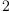(1)
Type: PositiveInteger?
fricas
macro ℒ == List
Type: Void
fricas
macro ℂ == CaleyDickson
Type: Void
fricas
macro ℚ == Expression Integer
Type: Void
fricas
𝐋 := ClosedLinearOperator(OVAR ['1,'2], ℚ)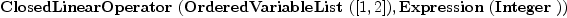(2)
Type: Type
fricas
𝐞:ℒ 𝐋      := basisOut()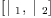(3)
Type: List(ClosedLinearOperator(OrderedVariableList([1,2]),Expression(Integer)))
fricas
𝐝:ℒ 𝐋      := basisIn()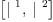(4)
Type: List(ClosedLinearOperator(OrderedVariableList([1,2]),Expression(Integer)))
fricas
I:𝐋:=   -- identity for composition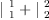(5)
Type: ClosedLinearOperator(OrderedVariableList([1,2]),Expression(Integer))
fricas
X:𝐋:=[2,1] -- twist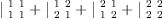(6)
Type: ClosedLinearOperator(OrderedVariableList([1,2]),Expression(Integer))
fricas
V:𝐋:=ev(1) -- evaluation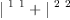(7)
Type: ClosedLinearOperator(OrderedVariableList([1,2]),Expression(Integer))
fricas
Λ:𝐋:=co(1) -- co-evaluation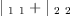(8)
Type: ClosedLinearOperator(OrderedVariableList([1,2]),Expression(Integer))
fricas
!:𝐋:=[-1]  -- color change 1 -> 1*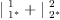(9)
Type: ClosedLinearOperator(OrderedVariableList([1,2]),Expression(Integer))
fricas
$:𝐋:=dagger ! -- 1* -> 1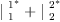(10) Type: ClosedLinearOperator(OrderedVariableList([1,2]),Expression(Integer)) fricas J:𝐋:=$/!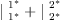(11)
Type: ClosedLinearOperator(OrderedVariableList([1,2]),Expression(Integer))
fricas
test(!/$=I)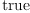(12) Type: Boolean fricas equate(eq)==map((x,y)+->(x=y),ravel lhs eq, ravel rhs eq); Type: Void We want to be able to implement linear operators with two "colors" like the following: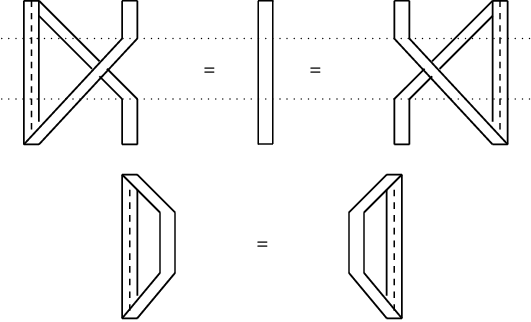An example starting with Complex Algebra The basis consists of the real and imaginary units. We use complex multiplication to form the "multiplication table" as a matrix. Then the structure constants can be obtained by dividing each matrix entry by the list of basis vectors. Split-complex can be specified by Caley-Dickson parameter (q0 = -1) fricas --q:=1 -- split-complex q:=sp('i,)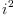(13) Type: Symbol fricas QQ := ℂ(ℚ,'i,q); Type: Type Basis: Each B.i is a complex number fricas B:ℒ QQ := map(x +-> hyper x,1$SQMATRIX(dim,ℚ)::ℒ ℒ ℚ)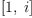(14)
Type: List(CaleyDickson(Expression(Integer),i,i[;2]))
fricas
-- Multiplication table:
M:Matrix QQ := matrix Ξ(Ξ(B.i*B.j, i,1..dim), j,1..dim)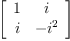(15)
Type: Matrix(CaleyDickson(Expression(Integer),i,i[;2]))
fricas
-- Function to divide the matrix entries by a basis element
S(y) == map(x +-> real real(x/y),M)
Type: Void
fricas
-- The result is a nested list
ѕ :=map(S,B)::ℒ ℒ ℒ ℚ
fricas
Compiling function S with type CaleyDickson(Expression(Integer),i,i[
;2]) -> Matrix(Expression(Integer))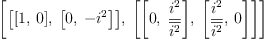(16)
Type: List(List(List(Expression(Integer))))
fricas
-- structure constants form a tensor operator
Y := Σ(Σ(Σ(ѕ(i)(k)(j)*𝐞.i*𝐝.j*($/𝐝.k), i,1..dim), j,1..dim), k,1..dim)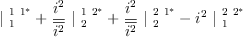(17) Type: ClosedLinearOperator(OrderedVariableList([1,2]),Expression(Integer)) fricas arity Y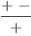(18) Type: ClosedProp?(ClosedLinearOperator(OrderedVariableList([1,2]),Expression(Integer))) fricas Y! := (I,!)/Y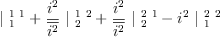(19) Type: ClosedLinearOperator(OrderedVariableList([1,2]),Expression(Integer)) fricas matrix Ξ(Ξ((𝐞.i*𝐞.j)/Y!, i,1..dim), j,1..dim)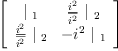(20) Type: Matrix(ClosedLinearOperator(OrderedVariableList([1,2]),Expression(Integer))) Multiplication of arbitrary quaternions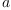and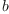fricas a:=Σ(sb('a,[i])*𝐞.i, i,1..dim)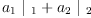(21) Type: ClosedLinearOperator(OrderedVariableList([1,2]),Expression(Integer)) fricas b:=Σ(sb('b,[i])*𝐞.i, i,1..dim)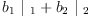(22) Type: ClosedLinearOperator(OrderedVariableList([1,2]),Expression(Integer)) fricas (a,b)/Y!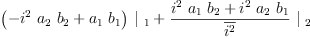(23) Type: ClosedLinearOperator(OrderedVariableList([1,2]),Expression(Integer)) Multiplication is Associative fricas test( ( Y! J ) / _ ( Y ) = _ ( I Y ) / _ ( Y! ) )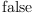(24) Type: Boolean A scalar product is denoted by the (2,0)-tensor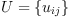fricas U:=Σ(Σ(script('u,[[],[i,j]])*𝐝.i*($/𝐝.j), i,1..dim), j,1..dim)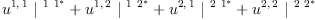(25)
Type: ClosedLinearOperator(OrderedVariableList([1,2]),Expression(Integer))
fricas
arity U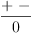(26)
Type: ClosedProp?(ClosedLinearOperator(OrderedVariableList([1,2]),Expression(Integer)))

## Definition 1

We say that the scalar product is associative if the tensor equation holds:

    Y   =   Y
U     U


In other words, if the (3,0)-tensor: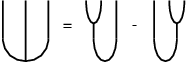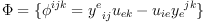(27)
(three-point function) is zero.

How should we color this?

 Subject:   Be Bold !! ( 14 subscribers )